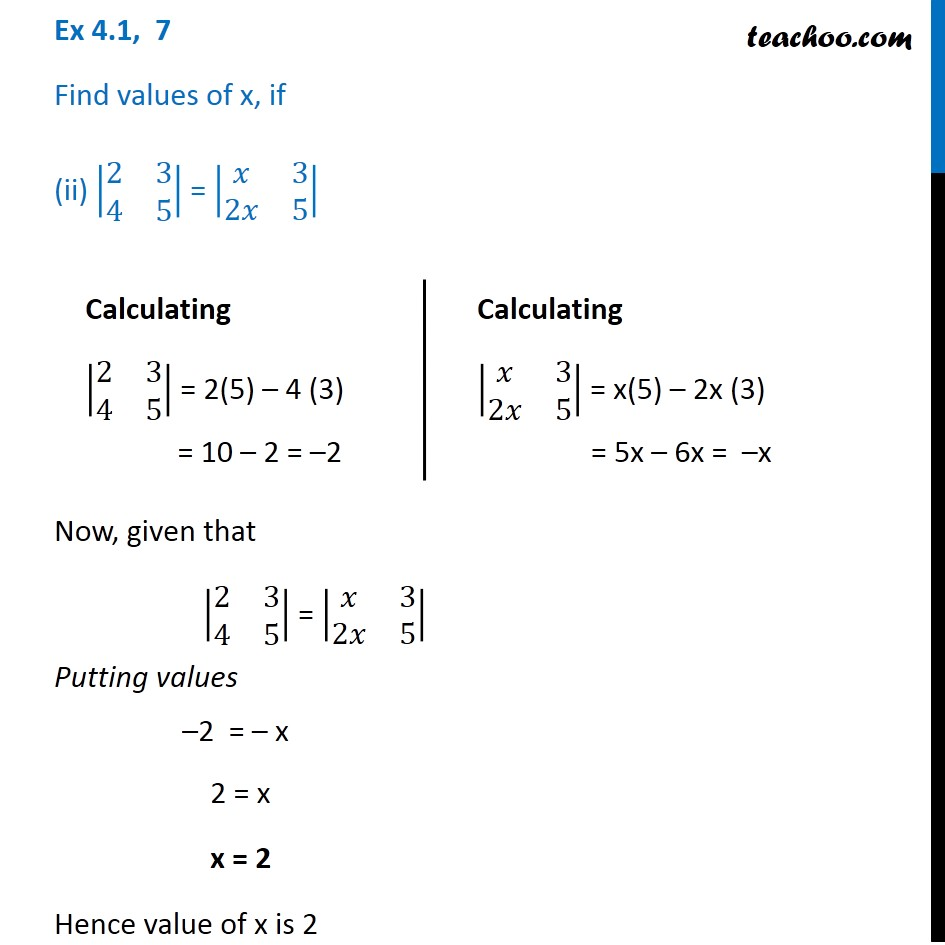1. Chapter 4 Class 12 Determinants (Term 1)
2. Serial order wise
3. Ex 4.1

Transcript

Ex 4.1, 7 Find values of x, if (ii) |■8(2&3@4&5)| = |■8(𝑥&3@2𝑥&5)| Calculating |■8(2&3@4&5)| = 2(5) – 4 (3) = 10 – 2 = –2 Calculating |■8(𝑥&3@2𝑥&5)| = x(5) – 2x (3) = 5x – 6x = –x Now, given that |■8(2&3@4&5)| = |■8(𝑥&3@2𝑥&5)| Putting values –2 = – x 2 = x x = 2 Hence value of x is 2